# Review of Propositional Logic Syntax Propositional variables x

• Slides: 17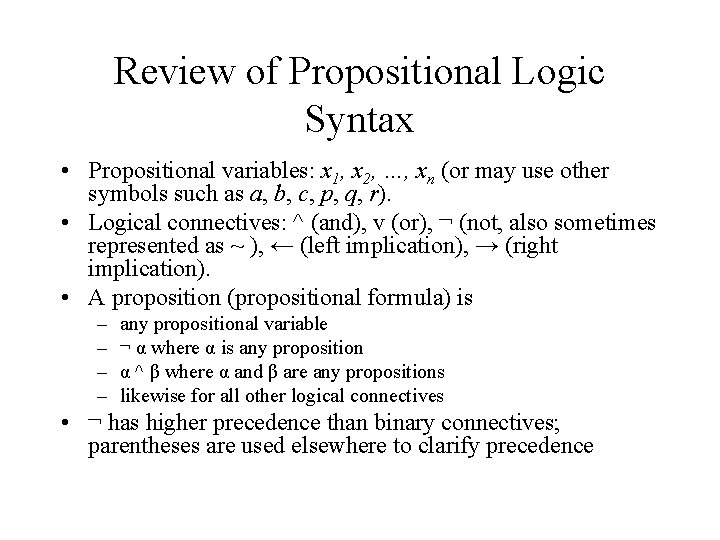Review of Propositional Logic Syntax • Propositional variables: x 1, x 2, …, xn (or may use other symbols such as a, b, c, p, q, r). • Logical connectives: ^ (and), v (or), ¬ (not, also sometimes represented as ~ ), ← (left implication), → (right implication). • A proposition (propositional formula) is – – any propositional variable ¬ α where α is any proposition α ^ β where α and β are any propositions likewise for all other logical connectives • ¬ has higher precedence than binary connectives; parentheses are used elsewhere to clarify precedence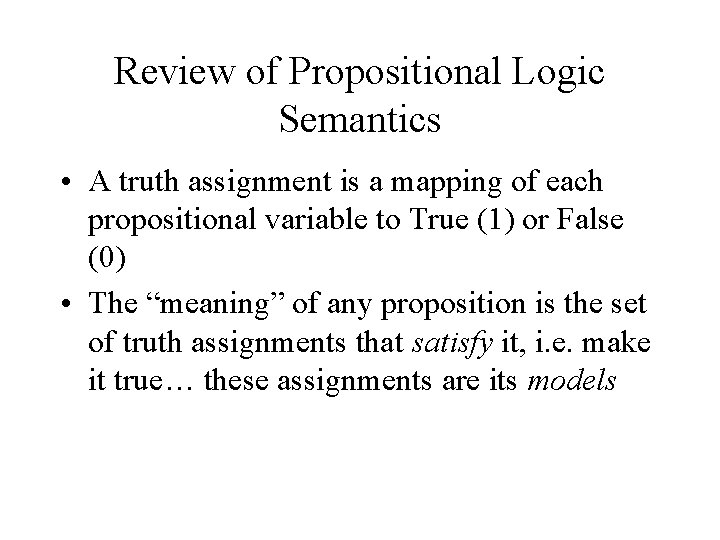Review of Propositional Logic Semantics • A truth assignment is a mapping of each propositional variable to True (1) or False (0) • The “meaning” of any proposition is the set of truth assignments that satisfy it, i. e. make it true… these assignments are its modelsSatisfaction • A truth assignment A satisfies a proposition iff (if and only if): – The proposition is a variable that A maps to True – The proposition is of the form ¬ α and A does not satisfy α – The proposition is of the form • α ^ β and A satisfies α and β • α v β and A satisfies α or β • α → β and if A satisfies α then it satisfies β (i. e. , A satisfies β or does not satisfy α, possibly both)Truth Tables • A truth table for a propositional formula shows all truth assignments for the variables in the formula, and the truth of the formula under each assignment. • An example: p 0 q 0 r 0 (pvq)→r 1 0 0 0 1 1 0 0 1 1 1 0 1 0 1 0 1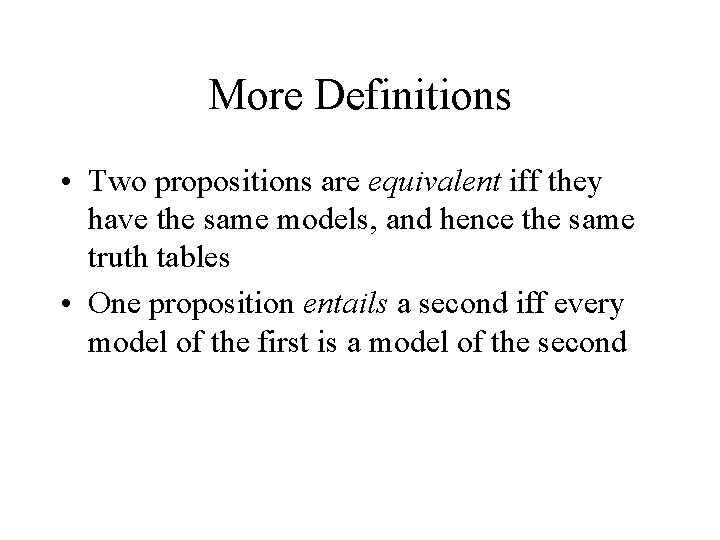More Definitions • Two propositions are equivalent iff they have the same models, and hence the same truth tables • One proposition entails a second iff every model of the first is a model of the secondSome Equivalences • • ¬¬α=α (α → β) = (¬α v β) De. Morgan’s Law: ¬(α ^ β) = (¬α v ¬β) Distributivity of ^ over v: α ^ (β v γ) = (α ^ β) v (α ^ γ) • Can show analogous equivalences for distributivity of v over ^ and for commutativity and associativity of ^ and v • Exercise: Show these using truth tables and also show you can swap ^ and v in De. Morgan’s Law.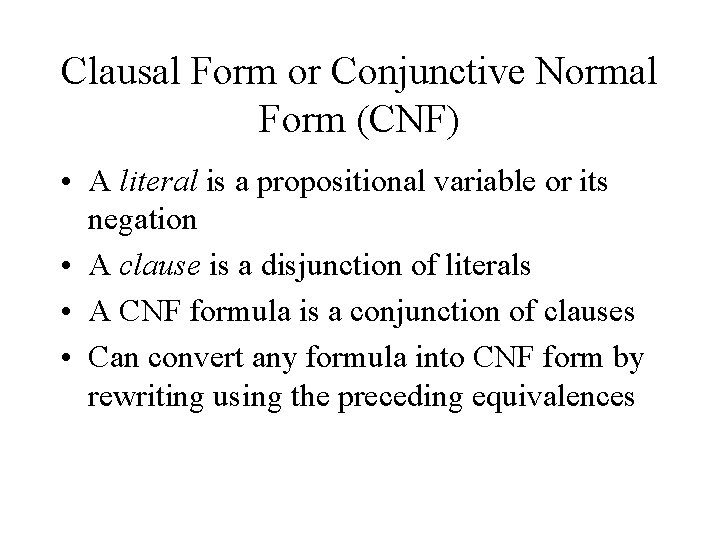Clausal Form or Conjunctive Normal Form (CNF) • A literal is a propositional variable or its negation • A clause is a disjunction of literals • A CNF formula is a conjunction of clauses • Can convert any formula into CNF form by rewriting using the preceding equivalences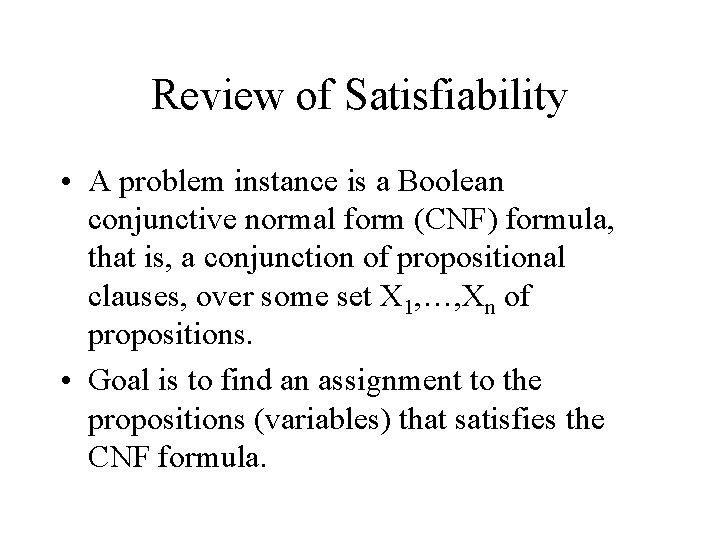Review of Satisfiability • A problem instance is a Boolean conjunctive normal form (CNF) formula, that is, a conjunction of propositional clauses, over some set X 1, …, Xn of propositions. • Goal is to find an assignment to the propositions (variables) that satisfies the CNF formula.Satisfiability Review (Continued) • Satisfiability is important for several reasons, including – It is at the foundation of NP-completeness – It’s the canonical example of constraint satisfaction problems (CSPs) – Many interesting tasks, including planning tasks, can be encoded as satisfiability problems. • Broadly speaking, CSPs grow easier withSatisfiability (Continued) • (Continued)… more variables but harder with more constraints. In the case of satisfiability, each clause is a constraint. • Kautz, Levesque, Mitchell, and Selman showed that the critical measure of hardness of satisfiability is the fraction of the number of clauses over the number of variables. For a large fraction, it’s almost always easy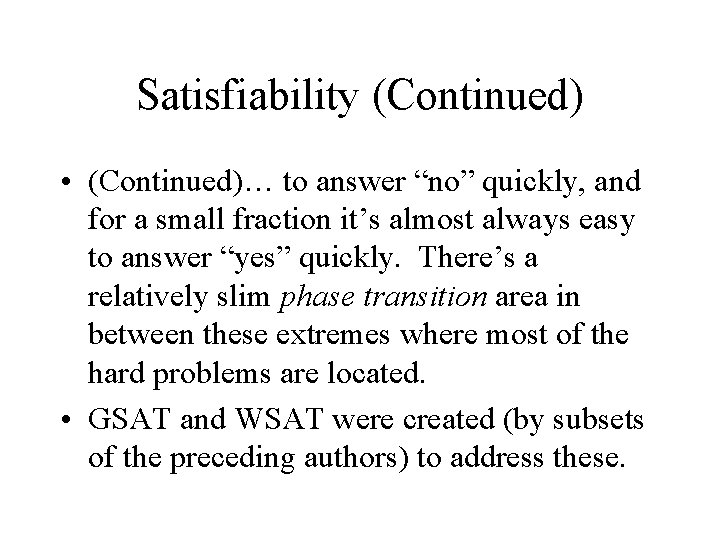Satisfiability (Continued) • (Continued)… to answer “no” quickly, and for a small fraction it’s almost always easy to answer “yes” quickly. There’s a relatively slim phase transition area in between these extremes where most of the hard problems are located. • GSAT and WSAT were created (by subsets of the preceding authors) to address these.GSAT • Input: CNF formula and integers Max_flips (e. g. 100) and Max_climbs (e. g. 20). • Output: Yes (satisfiable) or No (couldn’t find a satisfying assignment). Might also output the best assignment found. • Assignments are scored by the number of clauses they satisfy. • GSAT performs a beam search with random restarts and beam size set to 1.GSAT Algorithm • For i from 1 to Max_Climbs: – Randomly draw a truth assignment over the variables in the CNF formula (e. g. flip a coin for each variable to decide whether to make it 0 or 1 -- in practice, use pseudo-random number). If assignment satisfies formula, return “Yes”. – For j from 1 to Max_Flips: • For each variable, calculate the score of the truth assignment that results when we flip the value of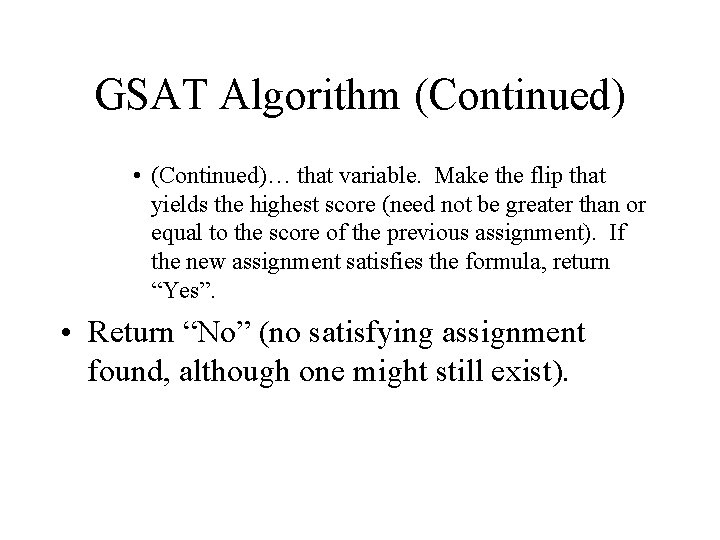GSAT Algorithm (Continued) • (Continued)… that variable. Make the flip that yields the highest score (need not be greater than or equal to the score of the previous assignment). If the new assignment satisfies the formula, return “Yes”. • Return “No” (no satisfying assignment found, although one might still exist).Key Points about GSAT • Cannot tell us a formula is unsatisfiable (but we can just run propositional resolution in parallel). • Random re-starts help us find multiple local optima -- the hope is that one will be global. • “Sideways” (or even “downward”) moves help us get off a plateau -- can bounce us off a local optimum. Significant practical advance over standard greedy approach.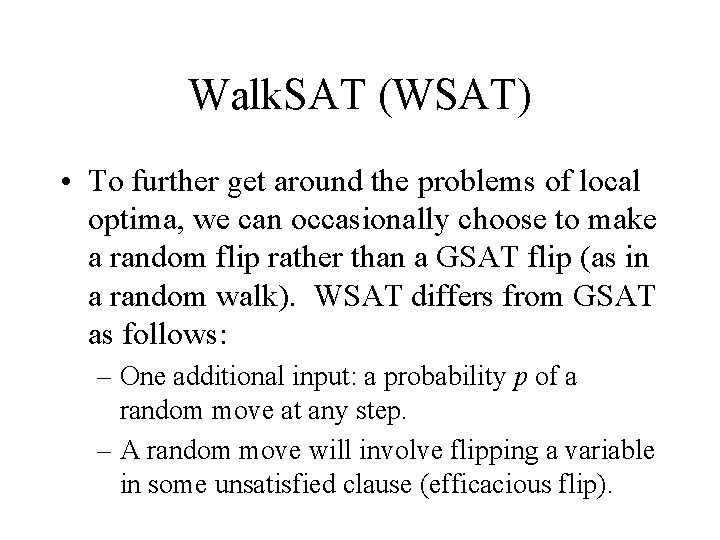Walk. SAT (WSAT) • To further get around the problems of local optima, we can occasionally choose to make a random flip rather than a GSAT flip (as in a random walk). WSAT differs from GSAT as follows: – One additional input: a probability p of a random move at any step. – A random move will involve flipping a variable in some unsatisfied clause (efficacious flip).WSAT (Continued) – (Continued)… This change will satisfy some currently unsatisfied clause (even if the net result of the flip is a decrease in score). – For each move, draw a pseudo-random number between 0 and 1. If less than p, make a random move; otherwise, make a GSAT move. • WSAT outperforms GSAT, Simulated Annealing, and a Genetic Algorithm on random trials.